# Title 40

## SECTION 1065.650

### 1065.650 Emission calculations.

§ 1065.650 Emission calculations.

(a) General. Calculate brake-specific emissions over each applicable duty cycle or test interval. For test intervals with zero work (or power), calculate the emission mass (or mass rate), but do not calculate brake-specific emissions. For duty cycles with multiple test intervals, refer to the standard-setting part for calculations you need to determine a composite result, such as a calculation that weights and sums the results of individual test intervals in a duty cycle. If the standard-setting part does not include those calculations, use the equations in paragraph (g) of this section. This section is written based on rectangular integration, where each indexed value (i.e., “i”) represents (or approximates) the mean value of the parameter for its respective time interval, delta-t. You may also integrate continuous signals using trapezoidal integration consistent with good engineering judgment.

(b) Brake-specific emissions over a test interval. We specify three alternative ways to calculate brake-specific emissions over a test interval, as follows:

(1) For any testing, you may calculate the total mass of emissions, as described in paragraph (c) of this section, and divide it by the total work generated over the test interval, as described in paragraph (d) of this section, using the following equation: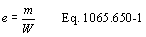Example: mNOx = 64.975 g W = 25.783 kW · hr eNOx = 64.975/25.783 eNOx = 2.520 g/(kW · hr)

(2) For discrete-mode steady-state testing, you may calculate the brake-specific emissions over a test interval using the ratio of emission mass rate to power, as described in paragraph (e) of this section, using the following equation: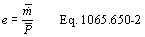(3) For field testing, you may calculate the ratio of total mass to total work, where these individual values are determined as described in paragraph (f) of this section. You may also use this approach for laboratory testing, consistent with good engineering judgment. Good engineering judgment dictates that this method not be used if there are any work flow paths described in § 1065.210 that cross the system boundary, other than the primary output shaft (crankshaft). This is a special case in which you use a signal linearly proportional to raw exhaust molar flow rate to determine a value proportional to total emissions. You then use the same linearly proportional signal to determine total work using a chemical balance of fuel, intake air, and exhaust as described in § 1065.655, plus information about your engine's brake-specific fuel consumption. Under this method, flow meters need not meet accuracy specifications, but they must meet the applicable linearity and repeatability specifications in subpart D or subpart J of this part. The result is a brake-specific emission value calculated as follows: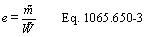Example: m = 805.5 g W = 52.102 kW · hr eCO = 805.5/52.102 eCO = 2.520 g/(kW · hr)

(c) Total mass of emissions over a test interval. To calculate the total mass of an emission, multiply a concentration by its respective flow. For all systems, make preliminary calculations as described in paragraph (c)(1) of this section to correct concentrations. Next, use the method in paragraphs (c)(2) through (4) of this section that is appropriate for your system. Finally, if necessary, calculate the mass of NMHC as described in paragraph (c)(5) of this section for all systems. Calculate the total mass of emissions as follows:

(1) Concentration corrections. Perform the following sequence of preliminary calculations on recorded concentrations:

(i) Correct all gaseous emission analyzer concentration readings, including continuous readings, sample bag readings, and dilution air background readings, for drift as described in § 1065.672. Note that you must omit this step where brake-specific emissions are calculated without the drift correction for performing the drift validation according to § 1065.550(b). When applying the initial THC and CH4 contamination readings according to § 1065.520(f), use the same values for both sets of calculations. You may also use as-measured values in the initial set of calculations and corrected values in the drift-corrected set of calculations as described in § 1065.520(f)(7).

(ii) Correct all THC and CH4 concentrations for initial contamination as described in § 1065.660(a), including continuous readings, sample bags readings, and dilution air background readings.

(iii) Correct all concentrations measured on a “dry” basis to a “wet” basis, including dilution air background concentrations, as described in § 1065.659.

(iv) Calculate all NMHC and CH4 concentrations, including dilution air background concentrations, as described in § 1065.660.

(v) For emission testing with an oxygenated fuel, calculate any HC concentrations, including dilution air background concentrations, as described in § 1065.665. See subpart I of this part for testing with oxygenated fuels.

(vi) Correct all the NOX concentrations, including dilution air background concentrations, for intake-air humidity as described in § 1065.670.

(2) Continuous sampling. For continuous sampling, you must frequently record a continuously updated concentration signal. You may measure this concentration from a changing flow rate or a constant flow rate (including discrete-mode steady-state testing), as follows:

(i) Varying flow rate. If you continuously sample from a changing exhaust flow rate, time align and then multiply concentration measurements by the flow rate from which you extracted it. Use good engineering judgment to time align flow and concentration data to match transformation time, t50, to within ±1 s. We consider the following to be examples of changing flows that require a continuous multiplication of concentration times molar flow rate: Raw exhaust, exhaust diluted with a constant flow rate of dilution air, and CVS dilution with a CVS flowmeter that does not have an upstream heat exchanger or electronic flow control. This multiplication results in the flow rate of the emission itself. Integrate the emission flow rate over a test interval to determine the total emission. If the total emission is a molar quantity, convert this quantity to a mass by multiplying it by its molar mass, M. The result is the mass of the emission, m. Calculate m for continuous sampling with variable flow using the following equations: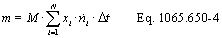Where: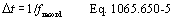Example: MNMHC = 13.875389 g/mol N = 1200 xNMHC1 = 84.5 µmol/mol = 84.5 · 10−6 mol/mol xNMHC2 = 86.0 µmol/mol = 86.0 · 10−6 mol/mol n exh1 = 2.876 mol/s n exh2 = 2.224 mol/s frecord = 1 Hz Using Eq. 1065.650-5, Δt = 1/1 = 1 s mNMHC = 13.875389 · (84.5 · 10−6 · 2.876 + 86.0 · 10−6 · 2.224 + ... + xNMHC1200 · n exh) · 1 mNMHC = 25.53 g

(ii) Constant flow rate. If you continuously sample from a constant exhaust flow rate, use the same emission calculations described in paragraph (c)(2)(i) of this section or calculate the mean or flow-weighted concentration recorded over the test interval and treat the mean as a batch sample, as described in paragraph (c)(3)(ii) of this section. We consider the following to be examples of constant exhaust flows: CVS diluted exhaust with a CVS flowmeter that has either an upstream heat exchanger, electronic flow control, or both.

(3) Batch sampling. For batch sampling, the concentration is a single value from a proportionally extracted batch sample (such as a bag, filter, impinger, or cartridge). In this case, multiply the mean concentration of the batch sample by the total flow from which the sample was extracted. You may calculate total flow by integrating a changing flow rate or by determining the mean of a constant flow rate, as follows:

(i) Varying flow rate. If you collect a batch sample from a changing exhaust flow rate, extract a sample proportional to the changing exhaust flow rate. We consider the following to be examples of changing flows that require proportional sampling: Raw exhaust, exhaust diluted with a constant flow rate of dilution air, and CVS dilution with a CVS flowmeter that does not have an upstream heat exchanger or electronic flow control. Integrate the flow rate over a test interval to determine the total flow from which you extracted the proportional sample. Multiply the mean concentration of the batch sample by the total flow from which the sample was extracted. If the total emission is a molar quantity, convert this quantity to a mass by multiplying it by its molar mass, M. The result is the mass of the emission, m. In the case of PM emissions, where the mean PM concentration is already in units of mass per mole of sample, M PM, simply multiply it by the total flow. The result is the total mass of PM, mPM. Calculate m for batch sampling with variable flow using the following equation: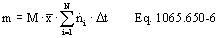Example: MNOx = 46.0055 g/mol N = 9000 x NOx = 85.6 µmol/mol = 85.6 · 10−6 mol/mol n dexh1 = 25.534 mol/s n dexh2 = 26.950 mol/s frecord = 5 Hz Using Eq. 1065.650-5, Δt = 1/5 = 0.2 mNOx = 46.0055 · 85.6 · 10−6 · (25.534 + 26.950 + ... + n exh9000) · 0.2 mNOx = 4.201 g

(ii) Constant flow rate. If you batch sample from a constant exhaust flow rate, extract a sample at a proportional or constant flow rate. We consider the following to be examples of constant exhaust flows: CVS diluted exhaust with a CVS flow meter that has either an upstream heat exchanger, electronic flow control, or both. Determine the mean molar flow rate from which you extracted the constant flow rate sample. Multiply the mean concentration of the batch sample by the mean molar flow rate of the exhaust from which the sample was extracted, and multiply the result by the time of the test interval. If the total emission is a molar quantity, convert this quantity to a mass by multiplying it by its molar mass, M. The result is the mass of the emission, m. In the case of PM emissions, where the mean PM concentration is already in units of mass per mole of sample, M PM, simply multiply it by the total flow, and the result is the total mass of PM, mPM. Calculate m for sampling with constant flow using the following equations: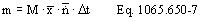and for PM or any other analysis of a batch sample that yields a mass per mole of sample,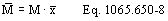Example: M PM = 144.0 µg/mol = 144.0 · 10−6 g/mol n dexh = 57.692 mol/s Δt = 1200 s mPM = 144.0 · 10−6 · 57.692 · 1200 mPM = 9.9692 g

(4) Additional provisions for diluted exhaust sampling; continuous or batch. The following additional provisions apply for sampling emissions from diluted exhaust:

(i) For sampling with a constant dilution ratio, DR, of diluted exhaust versus exhaust flow (e.g., secondary dilution for PM sampling), calculate m using the following equation: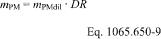Example: mPMdil = 6.853 g DR = 6:1 mPM = 6.853 · 6 mPM = 41.118 g

(ii) For continuous or batch sampling, you may measure background emissions in the dilution air. You may then subtract the measured background emissions, as described in § 1065.667.

(5) Mass of NMHC. Compare the corrected mass of NMHC to corrected mass of THC. If the corrected mass of NMHC is greater than 0.98 times the corrected mass of THC, take the corrected mass of NMHC to be 0.98 times the corrected mass of THC. If you omit the NMHC calculations as described in § 1065.660(b)(1), take the corrected mass of NMHC to be 0.98 times the corrected mass of THC.

(6) Mass of NMNEHC. If the test fuel has less than 0.010 mol/mol of ethane and you omit the NMNEHC calculations as described in § 1065.660(c)(1), take the corrected mass of NMNEHC to be 0.95 times the corrected mass of NMHC.

(d) Total work over a test interval. To calculate the total work from the engine over a test interval, add the total work from all the work paths described in § 1065.210 that cross the system boundary including electrical energy/work, mechanical shaft work, and fluid pumping work. For all work paths, except the engine's primary output shaft (crankshaft), the total work for the path over the test interval is the integration of the net work flow rate (power) out of the system boundary. When energy/work flows into the system boundary, this work flow rate signal becomes negative; in this case, include these negative work rate values in the integration to calculate total work from that work path. Some work paths may result in a negative total work. Include negative total work values from any work path in the calculated total work from the engine rather than setting the values to zero. The rest of this paragraph (d) describes how to calculate total work from the engine's primary output shaft over a test interval. Before integrating power on the engine's primary output shaft, adjust the speed and torque data for the time alignment used in § 1065.514(c). Any advance or delay used on the feedback signals for cycle validation must also be used for calculating work. Account for work of accessories according to § 1065.110. Exclude any work during cranking and starting. Exclude work during actual motoring operation (negative feedback torques), unless the engine was connected to one or more energy storage devices. Examples of such energy storage devices include hybrid powertrain batteries and hydraulic accumulators, like the ones illustrated in Figure 1 of § 1065.210. Exclude any work during reference zero-load idle periods (0% speed or idle speed with 0 N · m reference torque). Note, that there must be two consecutive reference zero load idle points to establish a period where this applies. Include work during idle points with simulated minimum torque such as Curb Idle Transmissions Torque (CITT) for automatic transmissions in “drive”. The work calculation method described in paragraphs (b)(1) through (7) of this section meets these requirements using rectangular integration. You may use other logic that gives equivalent results. For example, you may use a trapezoidal integration method as described in paragraph (b)(8) of this section.

(1) Time align the recorded feedback speed and torque values by the amount used in § 1065.514(c).

(2) Calculate shaft power at each point during the test interval by multiplying all the recorded feedback engine speeds by their respective feedback torques.

(3) Adjust (reduce) the shaft power values for accessories according to § 1065.110.

(4) Set all power values during any cranking or starting period to zero. See § 1065.525 for more information about engine cranking.

(5) Set all negative power values to zero, unless the engine was connected to one or more energy storage devices. If the engine was tested with an energy storage device, leave negative power values unaltered.

(6) Set all power values to zero during idle periods with a corresponding reference torque of 0 N · m.

(7) Integrate the resulting values for power over the test interval. Calculate total work as follows: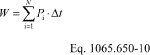Where: W = total work from the primary output shaft. Pi = instantaneous power from the primary output shaft over an interval i.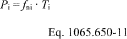Where: N = 9000 ƒn1 = 1800.2 r/min ƒn2 = 1805.8 r/min T1 = 177.23 N · m T2 = 175.00 N · m Crev = 2 · p rad/r Ct1 = 60 s/min Cp = 1000 (N · m · rad/s)/kW ƒrecord = 5 Hz Ct2 = 3600 s/hr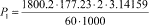P1 = 33.41 kW P2 = 33.09 kW Using Eq. 1065.650-5, Δt = 1/5 = 0.2 s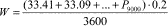W = 16.875 kW · hr

(8) You may use a trapezoidal integration method instead of the rectangular integration described in this paragraph (d). To do this, you must integrate the fraction of work between points where the torque is positive. You may assume that speed and torque are linear between data points. You may not set negative values to zero before running the integration.

(e) Steady-state mass rate divided by power. To determine steady-state brake-specific emissions for a test interval as described in paragraph (b)(2) of this section, calculate the mean steady-state mass rate of the emission, m , and the mean steady-state power, P as follows:

(1) To calculate m , multiply its mean concentration, x , by its corresponding mean molar flow rate, n . If the result is a molar flow rate, convert this quantity to a mass rate by multiplying it by its molar mass, M. The result is the mean mass rate of the emission, m . In the case of PM emissions, where the mean PM concentration is already in units of mass per mole of sample, M PM, simply multiply it by the mean molar flow rate, n . The result is the mass rate of PM, m PM. Calculate m using the following equation: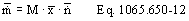(2) To calculate an engine's mean steady-state total power, P , add the mean steady-state power from all the work paths described in § 1065.210 that cross the system boundary including electrical power, mechanical shaft power, and fluid pumping power. For all work paths, except the engine's primary output shaft (crankshaft), the mean steady-state power over the test interval is the integration of the net work flow rate (power) out of the system boundary divided by the period of the test interval. When power flows into the system boundary, the power/work flow rate signal becomes negative; in this case, include these negative power/work rate values in the integration to calculate the mean power from that work path. Some work paths may result in a negative mean power. Include negative mean power values from any work path in the mean total power from the engine rather than setting these values to zero. The rest of this paragraph (e)(2) describes how to calculate the mean power from the engine's primary output shaft. Calculate P using Eq. 1065.650-13, noting that P , f n, and T refer to mean power, mean rotational shaft frequency, and mean torque from the primary output shaft. Account for the power of simulated accessories according to § 1065.110 (reducing the mean primary output shaft power or torque by the accessory power or torque). Set the power to zero during actual motoring operation (negative feedback torques), unless the engine was connected to one or more energy storage devices. Examples of such energy storage devices include hybrid powertrain batteries and hydraulic accumulators, like the ones illustrated in Figure 1 of § 1065.210. Set the power to zero for modes with a zero reference load (0 N·m reference torque or 0 kW reference power). Include power during idle modes with simulated minimum torque or power.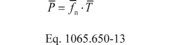(3) Divide emission mass rate by power to calculate a brake-specific emission result as described in paragraph (b)(2) of this section.

(4) The following example shows how to calculate mass of emissions using mean mass rate and mean power:

MCO = 28.0101 g/mol x CO = 12.00 mmol/mol = 0.01200 mol/mol n = 1.530 mol/s f n = 3584.5 r/min = 375.37 rad/s T = 121.50 N · m m = 28.0101 · 0.01200 · 1.530 m = 0.514 g/s = 1850.4 g/hr P = 121.5 · 375.37 P = 45607 W P = 45.607 kW eCO = 1850.4/45.61 eCO = 40.57 g/(kW · hr)

(f) Ratio of total mass of emissions to total work. To determine brake-specific emissions for a test interval as described in paragraph (b)(3) of this section, calculate a value proportional to the total mass of each emission. Divide each proportional value by a value that is similarly proportional to total work.

(1) Total mass. To determine a value proportional to the total mass of an emission, determine total mass as described in paragraph (c) of this section, except substitute for the molar flow rate, n , or the total flow, n, with a signal that is linearly proportional to molar flow rate, n , or linearly proportional to total flow, n as follows: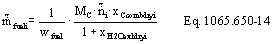(2) Total work. To calculate a value proportional to total work over a test interval, integrate a value that is proportional to power. Use information about the brake-specific fuel consumption of your engine, efuel, to convert a signal proportional to fuel flow rate to a signal proportional to power. To determine a signal proportional to fuel flow rate, divide a signal that is proportional to the mass rate of carbon products by the fraction of carbon in your fuel, wC. You may use a measured wC or you may use default values for a given fuel as described in § 1065.655(e). Calculate the mass rate of carbon from the amount of carbon and water in the exhaust, which you determine with a chemical balance of fuel, intake air, and exhaust as described in § 1065.655. In the chemical balance, you must use concentrations from the flow that generated the signal proportional to molar flow rate, n , in paragraph (e)(1) of this section. Calculate a value proportional to total work as follows: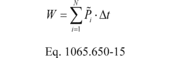Where: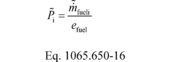(3) Brake-specific emissions. Divide the value proportional to total mass by the value proportional to total work to determine brake-specific emissions, as described in paragraph (b)(3) of this section.

(4) Example: The following example shows how to calculate mass of emissions using proportional values:

N = 3000 ƒrecord = 5 Hz efuel = 285 g/(kW·hr) wfuel = 0.869 g/g MC = 12.0107 g/mol n 1 = 3.922 mol/s = 14119.2 mol/hr xCcombdry1 = 91.634 mmol/mol = 0.091634 mol/mol xH2Oexh1 = 27.21 mmol/mol = 0.02721 mol/mol

Using Eq. 1065.650-5,

Δt = 0.2 s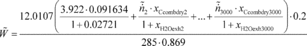W = 5.09 (kW·hr)

(g) Brake-specific emissions over a duty cycle with multiple test intervals. The standard-setting part may specify a duty cycle with multiple test intervals, such as with discrete-mode steady-state testing. Unless we specify otherwise, calculate composite brake-specific emissions over the duty cycle as described in this paragraph (g). If a measured mass (or mass rate) is negative, set it to zero for calculating composite brake-specific emissions, but leave it unchanged for drift validation. In the case of calculating composite brake-specific emissions relative to a combined emission standard (such as a NOX + NMHC standard), change any negative mass (or mass rate) values to zero for a particular pollutant before combining the values for the different pollutants.

(1) Use the following equation to calculate composite brake-specific emissions for duty cycles with multiple test intervals all with prescribed durations, such as cold-start and hot-start transient cycles: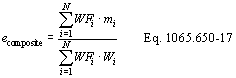Where: i = test interval number. N = number of test intervals. WF = weighting factor for the test interval as defined in the standard-setting part. m = mass of emissions over the test interval as determined in paragraph (c) of this section. W = total work from the engine over the test interval as determined in paragraph (d) of this section. Example: N = 2 WF1 = 0.1428 WF2 = 0.8572 m1 = 70.125 g m2 = 64.975 g W1 = 25.783 kW · hr W2 = 25.783 kW · hr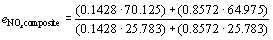eNOxcomposite = 2.548 g/kW · hr

(2) Calculate composite brake-specific emissions for duty cycles with multiple test intervals that allow use of varying duration, such as discrete-mode steady-state duty cycles, as follows:

(i) Use the following equation if you calculate brake-specific emissions over test intervals based on total mass and total work as described in paragraph (b)(1) of this section: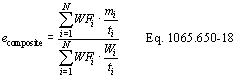Where: i = test interval number. N = number of test intervals. WF = weighting factor for the test interval as defined in the standard-setting part. m = mass of emissions over the test interval as determined in paragraph (c) of this section. W = total work from the engine over the test interval as determined in paragraph (d) of this section. t = duration of the test interval. Example: N = 2 WF1 = 0.85 WF2 = 0.15 m1 = 1.3753 g m2 = 0.4135 g t1 = 120 s t2 = 200 s W1 = 2.8375 kW · hr W2 = 0.0 kW · hr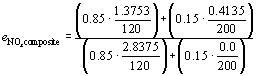eNOxcomposite = 0.5001 g/kW · hr

(ii) Use the following equation if you calculate brake-specific emissions over test intervals based on the ratio of mass rate to power as described in paragraph (b)(2) of this section: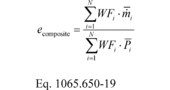Where: i = test interval number. N = number of test intervals. WF = weighting factor for the test interval as defined in the standard-setting part. m = mean steady-state mass rate of emissions over the test interval as determined in paragraph (e) of this section. P = mean steady-state power over the test interval as described in paragraph (e) of this section. Example: N = 2 WF1 = 0.85 WF2 = 0.15 m 1 = 2.25842 g/hr m 2 = 0.063443 g/hr P 1 = 4.5383 kW P 2 = 0.0 kW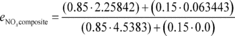eNOxcomposite = 0.5001 g/kW·hr

(h) Rounding. Round the final brake-specific emission values to be compared to the applicable standard only after all calculations are complete (including any drift correction, applicable deterioration factors, adjustment factors, and allowances) and the result is in g/(kW · hr) or units equivalent to the units of the standard, such as g/(hp · hr). See the definition of “Round” in § 1065.1001.

[73 FR 37328, June 30, 2008, as amended at 73 FR 59332, Oct. 8, 2008; 75 FR 23048, Apr. 30, 2010; 76 FR 57457, Sept. 15, 2011;79 FR 23799, Apr. 28, 2014; 80 FR 9118, Feb. 19, 2015; 81 FR 74180, Oct. 25, 2016]